Ex 7.1

Chapter 7 Class 9 Triangles
Serial order wise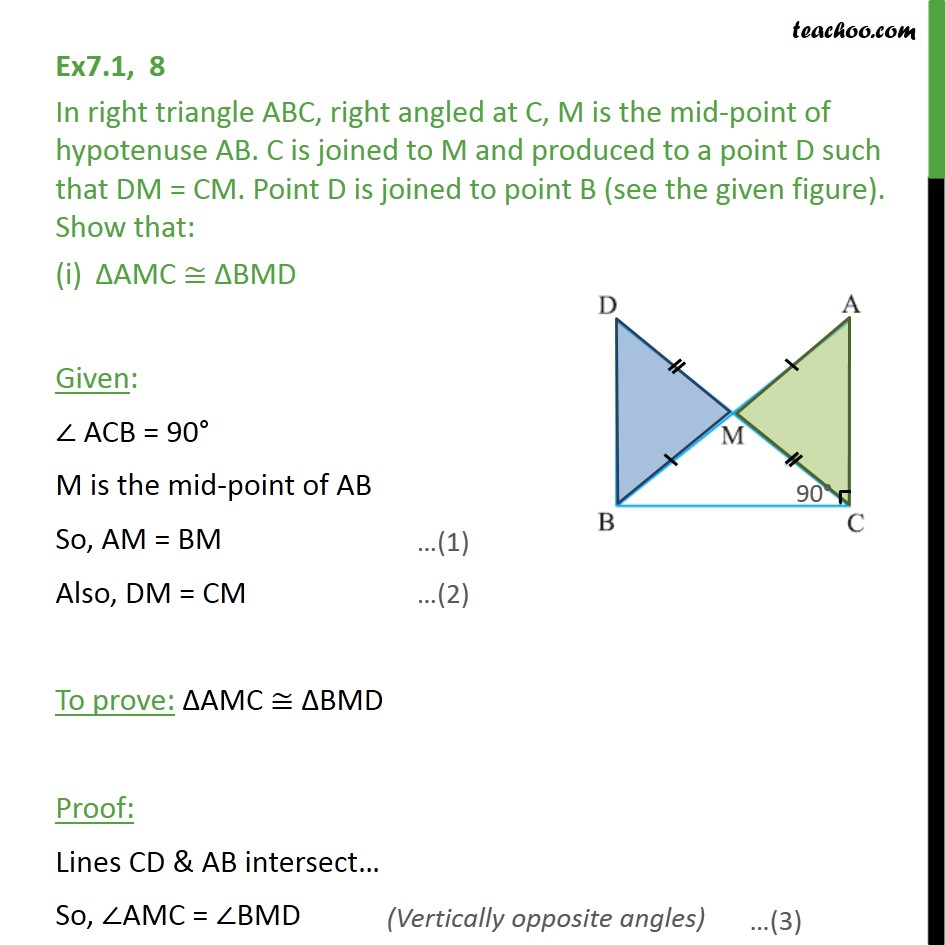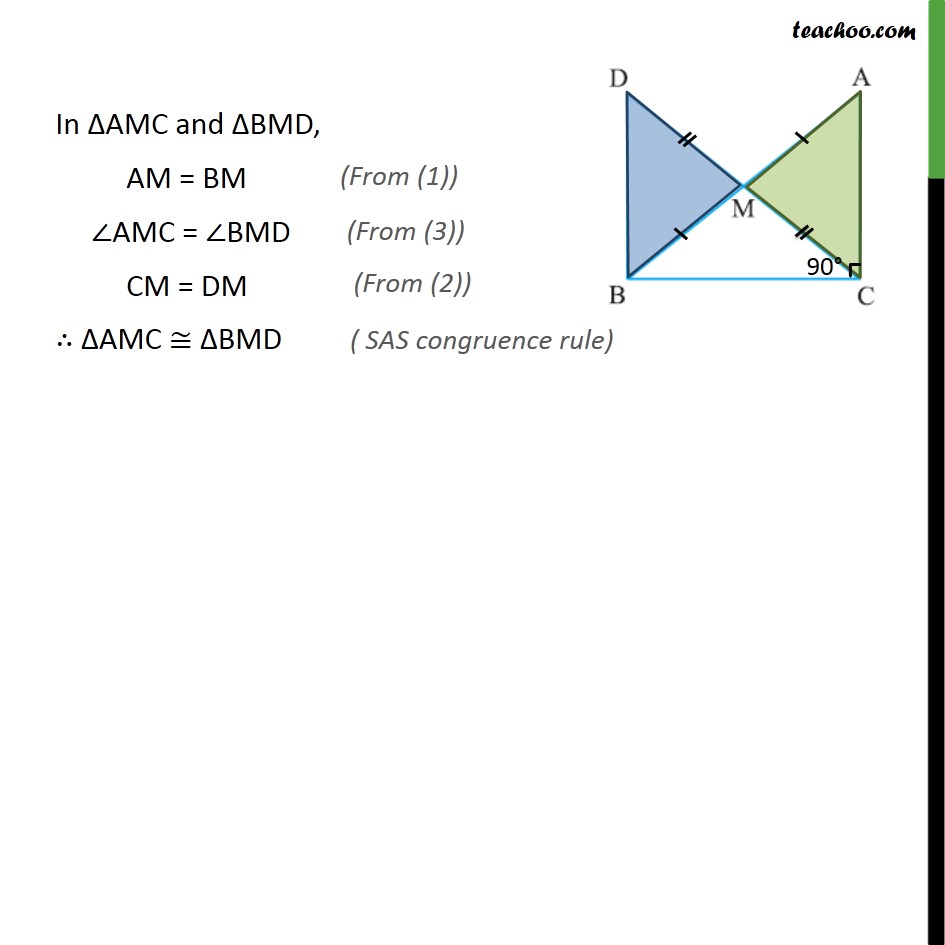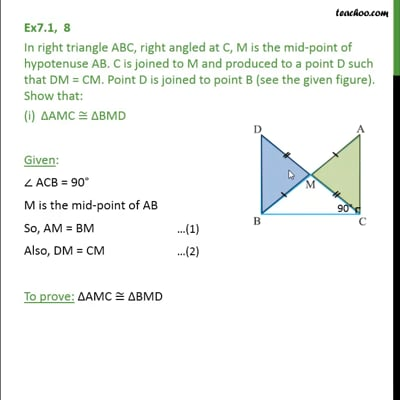This video is only available for Teachoo black users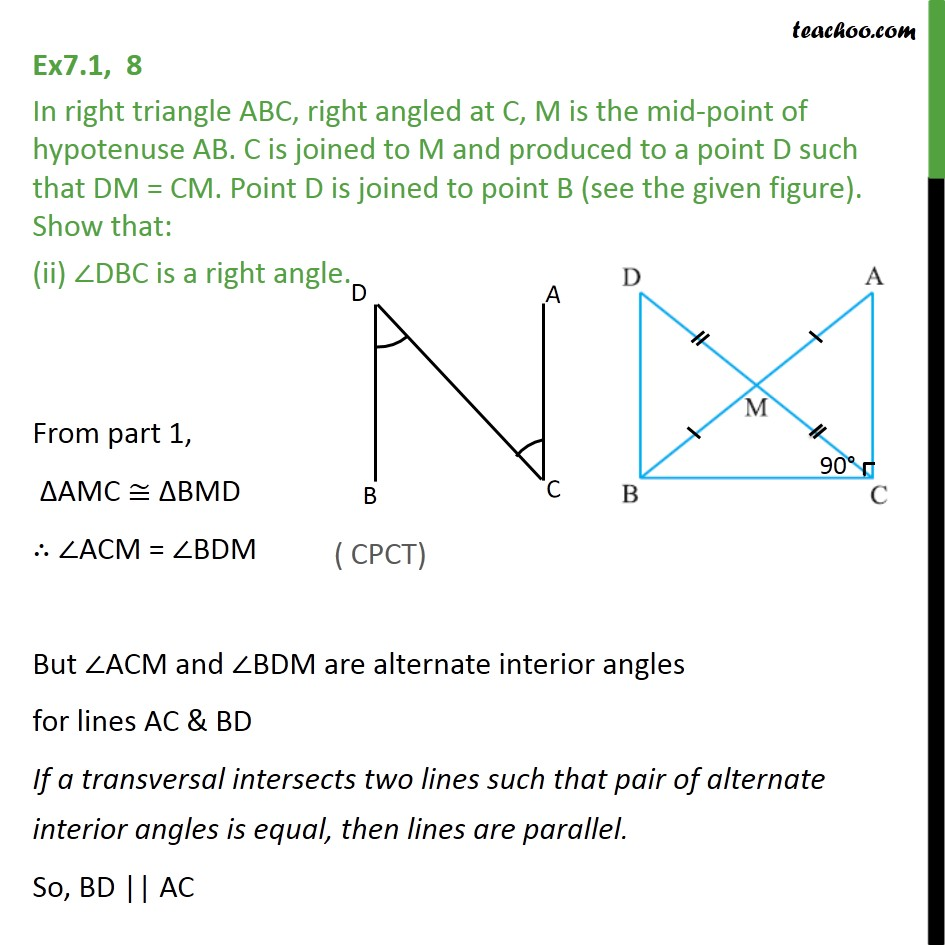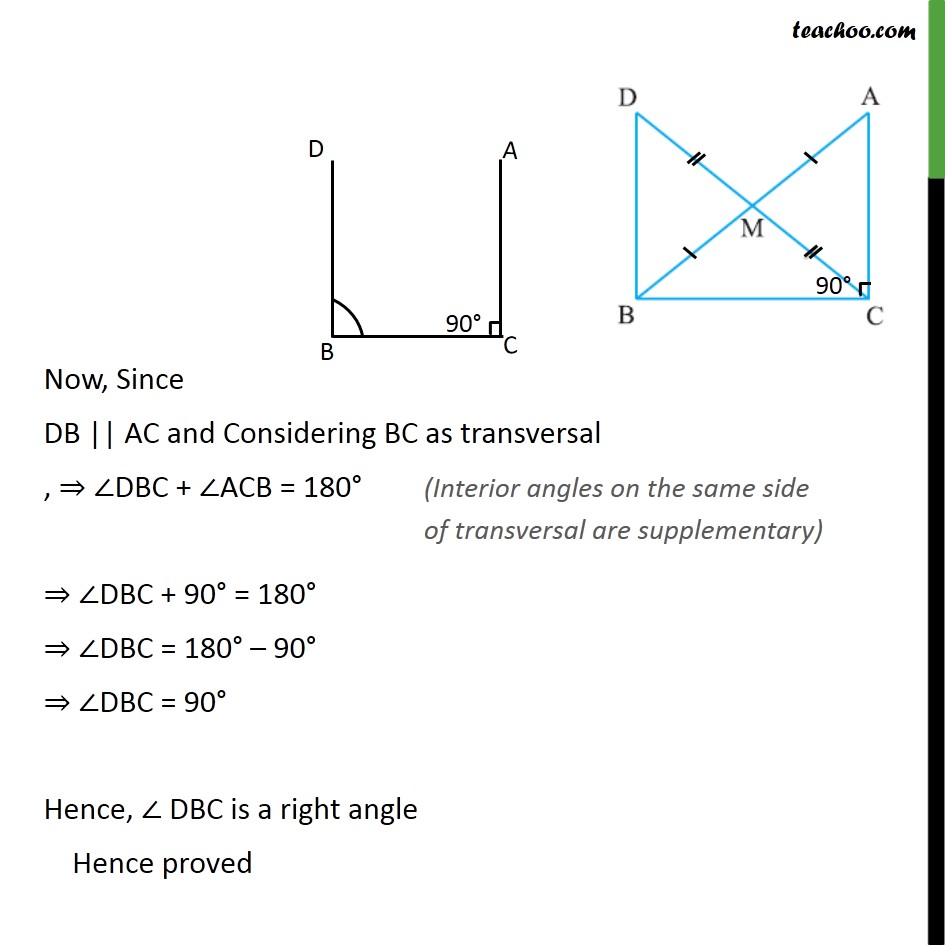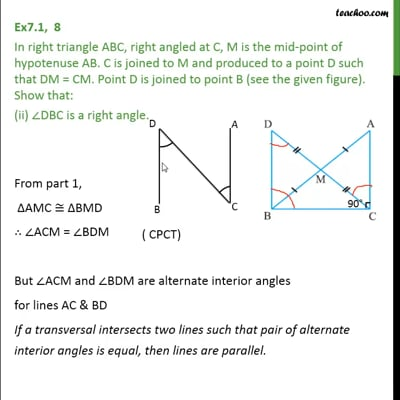This video is only available for Teachoo black users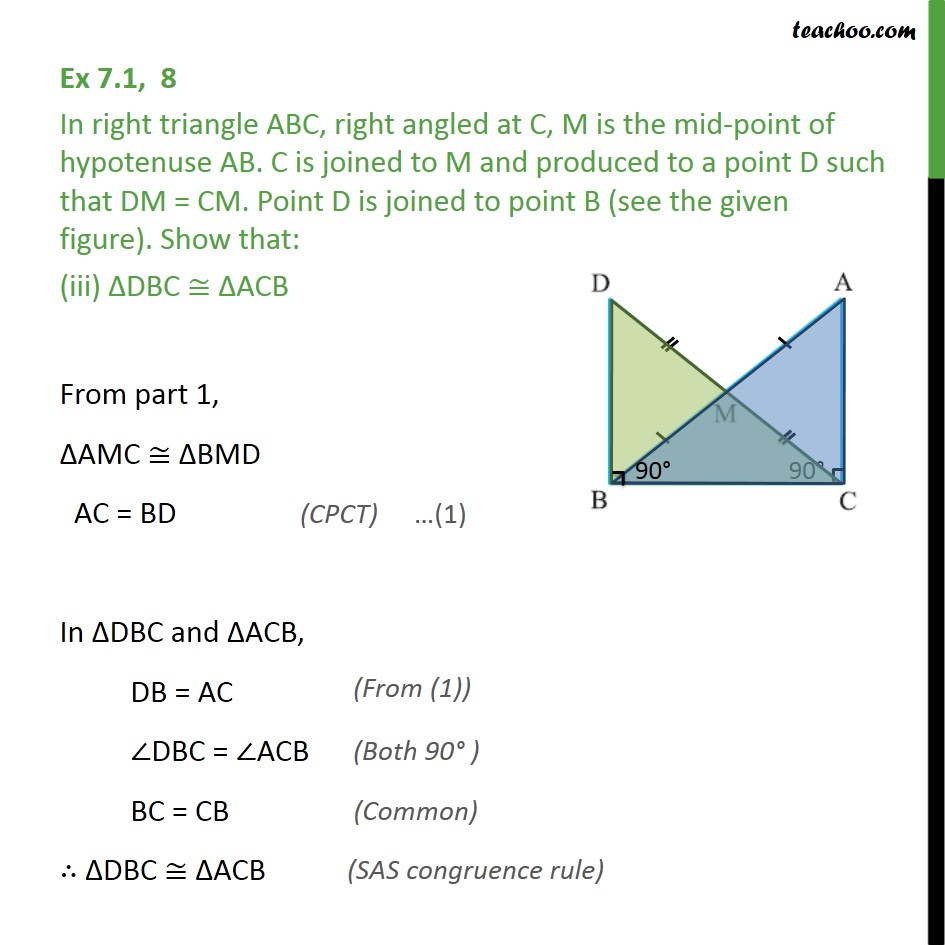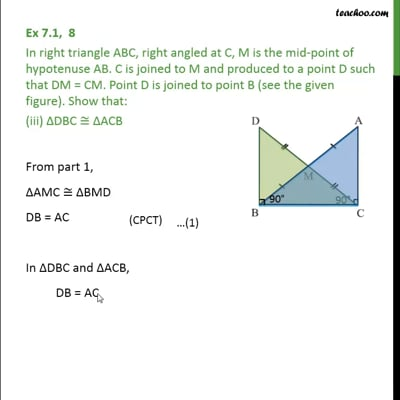This video is only available for Teachoo black users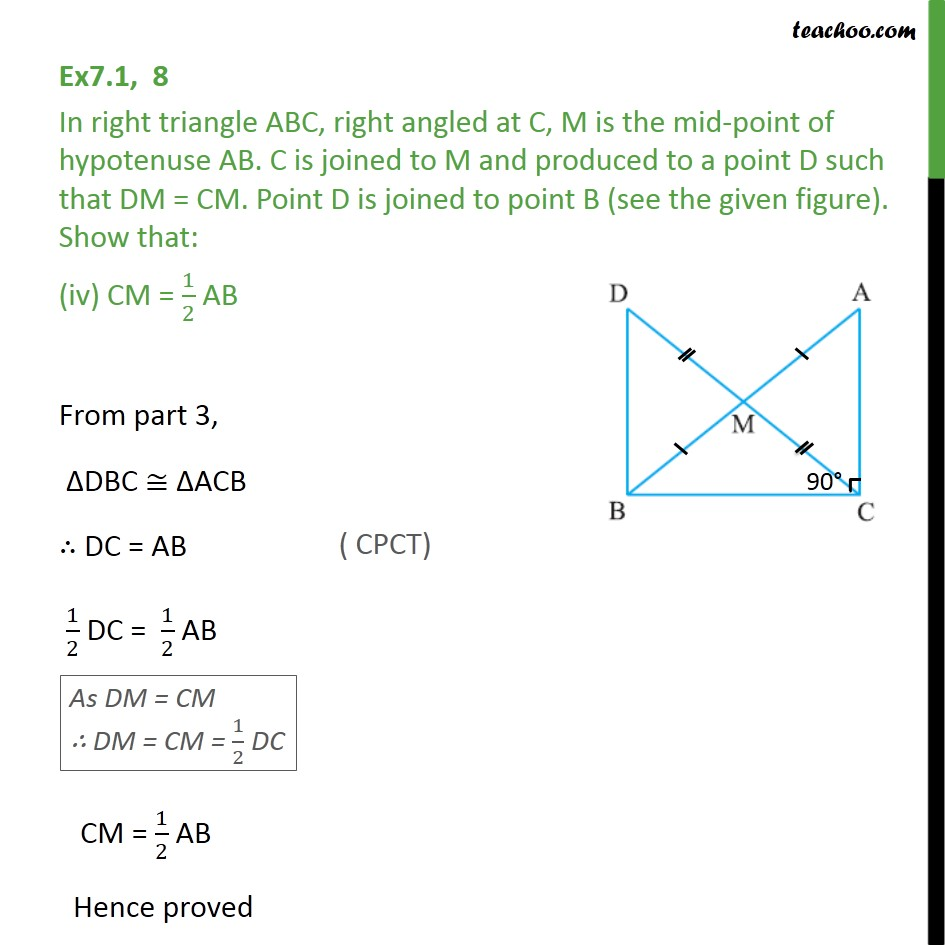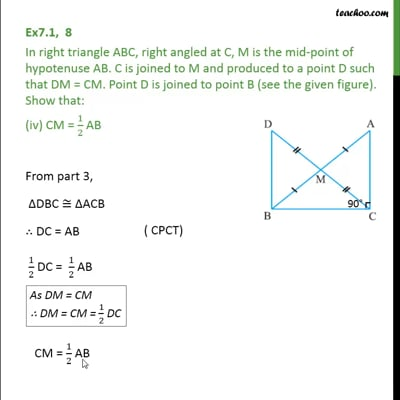This video is only available for Teachoo black users

Solve all your doubts with Teachoo Black (new monthly pack available now!)

### Transcript

Ex7.1, 8 In right triangle ABC, right angled at C, M is the mid-point of hypotenuse AB. C is joined to M and produced to a point D such that DM = CM. Point D is joined to point B (see the given figure). Show that: ΔAMC ≅ ΔBMD Given: ∠ ACB = 90° M is the mid-point of AB So, AM = BM Also, DM = CM To prove: ΔAMC ≅ ΔBMD Proof: Lines CD & AB intersect… So, ∠AMC = ∠BMD In ΔAMC and ΔBMD, AM = BM ∠AMC = ∠BMD CM = DM ∴ ΔAMC ≅ ΔBMD Ex7.1, 8 In right triangle ABC, right angled at C, M is the mid-point of hypotenuse AB. C is joined to M and produced to a point D such that DM = CM. Point D is joined to point B (see the given figure). Show that: (ii) ∠DBC is a right angle. From part 1, ΔAMC ≅ ΔBMD ∴ ∠ACM = ∠BDM But ∠ACM and ∠BDM are alternate interior angles for lines AC & BD If a transversal intersects two lines such that pair of alternate interior angles is equal, then lines are parallel. So, BD || AC Now, Since DB || AC and Considering BC as transversal , ⇒ ∠DBC + ∠ACB = 180° ⇒ ∠DBC + 90° = 180° ⇒ ∠DBC = 180° – 90° ⇒ ∠DBC = 90° Hence, ∠ DBC is a right angle Hence proved Ex 7.1, 8 In right triangle ABC, right angled at C, M is the mid-point of hypotenuse AB. C is joined to M and produced to a point D such that DM = CM. Point D is joined to point B (see the given figure). Show that: (iii) ΔDBC ≅ ΔACB From part 1, ΔAMC ≅ ΔBMD AC = BD In ΔDBC and ΔACB, DB = AC ∠DBC = ∠ACB BC = CB ∴ ΔDBC ≅ ΔACB Ex7.1, 8 In right triangle ABC, right angled at C, M is the mid-point of hypotenuse AB. C is joined to M and produced to a point D such that DM = CM. Point D is joined to point B (see the given figure). Show that: (iv) CM = 1/2 AB From part 3, ΔDBC ≅ ΔACB ∴ DC = AB 1/2 DC = 1/2 AB CM = 1/2 AB Hence proved